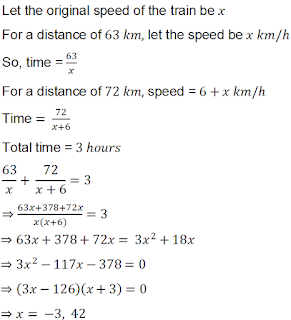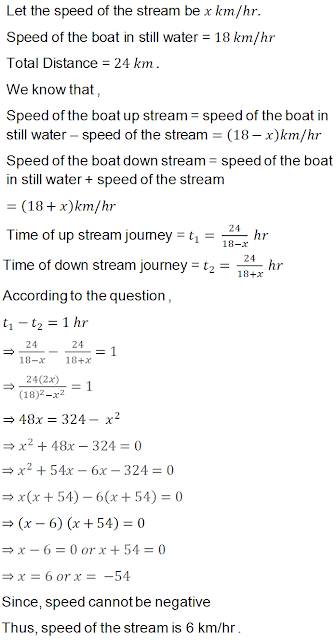## Chapter 8 Quadratic Equations R.D. Sharma Solutions for Class 10th Math Exercise 8.8

1. The speed of a boat in still water is 8 km/hr It can go 15 km upstream and 22 km downstream in 5 hours. Find the speed of the stream.

Solution2. A train , travelling at a uniform speed for 360 km, would have taken 48 minutes less to travel the same distance if its speed were 5 km / hr more. Find the original speed of the train.

Solution

Given,
A train is travelling at an unifrom speed for 360 km would have taken 48 min less to travel the same distance.
Let x be the original speed of the train.
time = distance / speed
A/q,

360/x = 360/(x + 5) + 48 / 60
⇒ 360/x - 360/(x + 5) = 4/5
⇒ 360[ 1/x - 1/( x +5) ] = 4/5
⇒ 450 [x + 5 - x  ] / x ( x +5) = 1
⇒ 2250 = x ( x +5)
⇒ x2 + 5x - 2250 = 0
⇒ x2 + 50x - 45x - 2250 = 0
⇒ x( x + 50) - 45( x + 50) = 0
⇒ (x - 45)( x + 50) = 0
x = 45 km /h

∴ The original speed of the train = 45 km /h.

3. A fast train takes one hour less than a slow train for a journey of 200 km. If the speed of the slow train is 10 km/hr less than that of the fast train, find the speed of the two trains.

Solution4. A passenger train takes one hour less for a journey of 150 km if its speed is increased by 5 km/hr from its usual speed. Find the usual speed of the train.

Solution5. The time taken by a person to cover 150 km was 2.5 hrs more than the time taken in the return journey. If he returned at a speed of 10 km/hr more than the speed of going, what was the speed per hour in each direction ?.

Solution6. A plane left 40 minutes late due to bad weather and in order to reach its destination, 1600 km away in time, it had to increase its speed by 400 km/hr from its usual speed. Find the usual speed of the plane.

Solution7. An aeroplane takes 1 hour less for a journey of 1200 km if its speed is increased by 100 km/hr from its usual speed. Find its usual speed.

Solution8. A train travels at a certain average speed for a distance 63 km and then travels a distance of 72 km at an average speed of 6 km/hr more than the original speed . If it takes 3 hours to complete total journey, what is its original as average speed ?

Solution9. A train covers a distance of 90 km at a uniform speed. Had the speed been 15 km/hour more, it would have taken 30 minutes less for the journey. Find the original speed of the train.

Solution10. A train travels 360 km at a uniform speed. If the speed had been 5 km/hr more, it would have taken 1 hour less for the same journey. Find the speed of the train.

Solution11. An express train takes 1 hour less than a passenger train to travel 132 km between Mysore and Bangalore (without taking into consideration the time they stop at intermediate stations). If the average speed of the express train is 11 kin/hr more than that of the passenger train, find the average speeds of the two trains.

Solution12. An aeroplane left 50 minutes later than its scheduled time, and in order to reach the destination, 1250 km away, in time, it had to increase its speed by 250 km/hr from its usual speed. Find its usual speed.

Solution13. While boarding an aeroplane , a passenger got hurt . The pilot showing promptness and concern, made arrangements to hospitalize the injured and so the plane started late by 30 minutes to reach the destination , 1500 km away in time , the pilot increased the speed by 100 km/hr . Find the original speed/hour of the plane .

Solution14. A motor boat whose speed in still water is 18/km/hr takes 1 hour more to go 24 km up stream that to return down stream to the same spot. Find the speed of the stream .

Solution